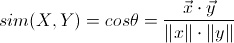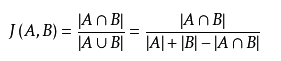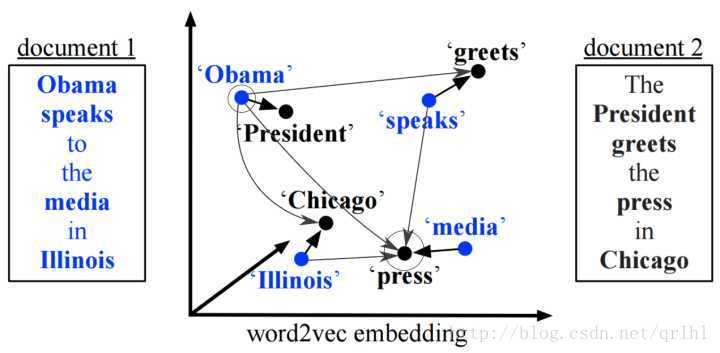# Week 6

## Vector Similarity Calculation

Posted by Stuart Chen on July 7, 2019

# The Week 6

This week we focus on two aspects.

First is the selection of the method for calculating the semantic vectors similarity.

Second is the experiment of doing benchmark evaluation in GERBIL-QA.

## 1. The Calculation Of Vectors Similarity

### 1.1 The Methods for Measuring the Vectors

Method 1: Cosine Similarity``````def cosine_distance(v1, v2):
if v1.all() and v2.all():
return np.dot(v1, v2) / (np.linalg.norm(v1) * np.linalg.norm(v2))
else:
return 0
``````

Method 2: Manhattan distance

Taxi geometry or Manhattan distance or grid line distance is a vocabulary created by Herman Minkowski, which is the geometric term used in the Euclidean geometric metric space to indicate two points. The sum of the absolute wheelbases on the standard coordinate system.

``````def manhattan_distance(v1, v2):  #
return np.sum(np.abs(v1 - v2))
``````

Method 3: Euclidean distance

Defined on two vectors (two points): the Euclidean distance between the points is:

``````def euclidean_distance(v1, v2):  #
return np.sqrt(np.sum(np.square(v1 - v2)))
``````

Method 4: Euclidean distance standardized

``````def euclidean_standardized_distance(v1, v2):  #
v1_v2 = np.vstack([v1, v2])
sk_v1_v2 = np.var(v1_v2, axis=0, ddof=1)
zero_bit = 0.000000001
distance = np.sqrt(((v1 - v2) ** 2 / (sk_v1_v2 + zero_bit * np.ones_like(sk_v1_v2))).sum())
return distance
``````

Method 5: Hamming Distance

In information theory, the Hamming distance between two strings of equal length is the number of positions at which the corresponding symbols are different.

``````def hamming_distance(v1, v2):
n = int(v1, 2) ^ int(v2, 2)
return bin(n & 0xffffffff).count('1')
``````

Method 6: Chebyshev distance

In mathematics, Chebyshev distance (or Tchebychev distance), maximum metric, or L∞ metric is a metric defined on a vector space where the distance between two vectors is the greatest of their differences along any coordinate dimension. It is named after Pafnuty Chebyshev.

``````def chebyshev_distance(v1, v2):  #
return np.max(np.abs(v1 - v2))
``````

Method 7: Minkowski distance

The Minkowski distance is a metric in a normed vector space which can be considered as a generalization of both the Euclidean distance and the Manhattan distance.

``````def minkowski_distance(v1, v2):  #
return np.sqrt(np.sum(np.square(v1 - v2)))
``````

Method 8: Mahalanobis distance

Defined on two vectors (two points), the two points are in the same distribution. The Mahalanobis distance between the points is:

``````def mahalanobis_distance(v1, v2):  #
X = np.vstack([v1, v2])
XT = X.T # numpy.ndarray.T
S = np.cov(X)  # Covariance matrix between two dimensions
try:
SI = np.linalg.inv(S)  # Inverse matrix of covariance matrix
except:
SI = np.zeros_like(S)
# The Mahalanobis distance calculates the distance between two samples. There are 10 samples in total, and there are a total of 45 distances.
n = XT.shape
distance_all = []
for i in range(0, n):
for j in range(i + 1, n):
delta = XT[i] - XT[j]
distance_1 = np.sqrt(np.dot(np.dot(delta, SI), delta.T))
distance_all.append(distance_1)
return np.sum(np.abs(distance_all))
``````

Method 9: Bray Curtis distance

In ecology and biology, the Bray–Curtis dissimilarity is a statistic used to quantify the compositional dissimilarity between two different sites, based on counts at each site.

``````def bray_curtis_distance(v1, v2):  #  Biological ecological distance
up_v1_v2 = np.sum(np.abs(v2 - v1))
down_v1_v2 = np.sum(v1) + np.sum(v2)
zero_bit = 0.000000001
return up_v1_v2 / (down_v1_v2 + zero_bit)
``````

Method 10: Pearson correlation

A Pearson correlation is a number between -1 and 1 that indicates the extent to which two variables are linearly related. The Pearson correlation is also known as the “product moment correlation coefficient” (PMCC) or simply “correlation”.

``````def pearson_correlation_distance(v1, v2):  #
v1_v2 = np.vstack([v1, v2])
return np.corrcoef(v1_v2)
``````

Method 11: Jaccard similarity coefficient

The Jaccard index, also known as Intersection over Union and the Jaccard similarity coefficient (originally given the French name coefficient de communauté by Paul Jaccard), is a statistic used for gauging the similarity and diversity of sample sets. If A and B are both empty, we define J(A,B) = 1.``````def jaccard_similarity_coefficient_distance(v1, v2):
v1 = np.asarray(v1)
v2 = np.asarray(v2)
up = np.double(np.bitwise_and((v1 != v2), np.bitwise_or(v1 != 0, v2 != 0)).sum())
zero_bit = 0.000000001
down = np.double(np.bitwise_or(v1 != 0, v2 != 0).sum() + zero_bit)
jaccard = up/down
return jaccard
``````

Method 12: Word Mover Distance

This is the method for comparing the sentences similarity.

The WMD model is based on the EMD (Earth Mover Distance) model. EMD is the same as Euclidean distance. They are definitions of distance metrics that can be used to measure the distance between two distributions. Its main application in the field of image processing and speech signal processing, WMD model is based on EMD, the scope of the model extends to the field of natural language processing. The detailed principle of EMD is not repeated here.

Matt et al. associate word embedding with EMD to measure document distance. The WMD (word mover’s distance) algorithm and WCD (word centroid distance) and RWMD (relaxed word mover’s distance) algorithms are proposed.

(1) The WMD algorithm uses NBOW (normalized bag-of-words, the normalized word bag model) to represent the distribution P. Where P1 represents the word itself, used to calculate the weight of the word in the current document, wherein the word i appears several times in the associated document, and the feature quantity of P1 is represented by the word vector of the word.

(2) The WMD algorithm uses a Word travel cost to calculate the similarity of the word i to the word j, that is, the Euclidean distance of the word vector of the word i and the word j, and the distance value is

C(i,j)=|(vecI -vecJ)|. Here, C(i,j) is seen as the price paid for converting the word i into the word j.

(3) The WMD algorithm uses the Document distance to represent the distance between documents.Define the matrix T here. Where Tij (Tij > = 0) indicates how much of the word i in the d document is converted into the word j in the d’ document. In order to ensure that the document d can be converted into the document d’, it is necessary to ensure that the sum of the quantities of all the words in the word d converted to d’ is di, that is, the same reason, it should also satisfy the word j converted into the d’ document in the d document. The total amount is dj, ie. We can define the distance between document d and d’ as the minimum cost of converting all words in d into words in d’.

``````def word_move_distance(model, sentence1_split, sentence2_split):  # WORD MOVER DISTANCE, it's the important one
# model = gensim.models.KeyedVectors.load_word2vec_format(word2_vec_path, unicode_errors='ignore', limit=None)  # ,binary=True)
# model.init_sims(replace=True)
distance = model.wmdistance(sentence1_split, sentence2_split)
return distance
``````

### 1.2 Choose Which Method:

First, we must liook at the constraints of what we want.

Second, it should be for what purpose.

To answer the first question, we must also keep in mind that we are using the metho on the word vectors.

To the second, we should focus on the distinguishability of the semantic meanings of two phrases in the form of GloVe vectors.

The Method 2(Manhattan distance) is not pertinent to our vector calculation, because we are not mearsuring a path.

The Method 3(Euclidean distance) is for measuring the spatial distance between two points, which might not reflect the similarity between two words.

The Method 5(Hamming Distance) is major in comparing the partial differences of two long string or documents, which might be not suitable for our phrases comparison.

The Method 7(Minkowski distance) is rather closer to the combination of Method 2 and Mehod 1, but is still more pertinent to the calculation of spatial distance.

Method 8(Mahalanobis distance), Method 9(Bray Curtis distance) and Method 10(Pearson correlation) are the metric based on statistic distribution, which might not be suitable for the GloVe vectors’ design ideas to represent the semantic meaning.

Then, the final candidate pool leaves only the method of cosine similarity and the method of jaccard similarity coefficient distance,

Let’s have the experiments:

• we randomly select a list of one hundred entities with related predicates from the generated training data, to see the precision that the two different mathod works
Method Accuracy
Cosine Similarity 53%
Jaccard Similarity 4%

• Analyses of The Result Let’s take an example: we used the natural language pair <”wife”, “Obama”> with expectation to get the entity pair <dbo:spouse, dbr:Barack_Obama>. So, did they succeed to get the dbo:spouse from “wife”?
Method Prediction Result
Cosine Similarity dbo:spouse, dbp:parents
Jaccard Similarity dbo:speaker

The Cosine method can catch more information in the vectors, while the Jaccard catches the distinguished 0-1 binary differences in each bit.

When looking into the two lists of scores that compared the similarity between the target phrase “wife” and the list of candidate strings, we found that: the scores of Jaccard method are mostly greater than 0.99, or otherwise 0; the scores of Jaccard method have an average approximate to 0.5.

And, according to a tutorial from Stanford ,

When utilities are more detailed ratings, the Jaccard distance loses important information.

Because Jaccard is mainly used to judge the similarity between sets, he can’t reflect more information like a matrix. A simple example might be, when I make a movie recommendation, I use the data of the user to calculate the similarity between the movies. With Jaccard, I can only use a single feature of the score that a watcher feedbacked, but in Cosine’s calculation, it can add every feature of the watcher’s rating information for the movie.

To sum up,

The Cosine similarity could be used to identify plagiarism, but will not be a good index to identify mirror sites on the internet. Whereas the Jaccard similarity will be a good index to identify mirror sites, but not so great at catching copy pasta plagiarism (within a larger document). 

 Jeffrey D. Ullman (2017) http://infolab.stanford.edu/~ullman/mmds/ch9.pdf

 OluwaYetty (2018) http://techinpink.com/2017/08/04/implementing-similarity-measures-cosine-similarity-versus-jaccard-similarity/